# Multiplication facts worksheets

## Times tables practice

These multiplication facts worksheets provide various exercise to help students gain fluency in the multiplication facts up to 12 x 12.

We also have multi-digit multiplication worksheets and thousands of math worksheets by grade level.

## Multiplication facts review

Multiplication facts review Tables

## Multiplication times tables (ordered)

Multiplication tables (ordered, 1-10)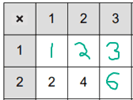Multiplication tables (ordered, 2-12)

## Multiplication times tables (random order)

Multiplication tables, 1-10, with hints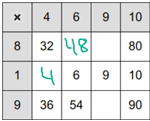Multiplication tables, 1-10
Multiplication tables, 2-12, with hints
Multiplication tables, 2-12

## Multiplication facts practice (vertical)

Multiplication facts 0-5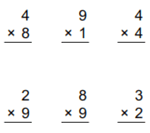Multiplication facts 0-7
Multiplication facts 1-10
Multiplication facts 2-12

## Multiplication facts practice (horizontal)

Multiplication facts 0-5 (horizontal)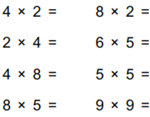Multiplication facts 0-7 (horizontal)
Multiplication facts 1-10 (horizontal)
Multiplication facts 2-12 (horizontal)

## Focus numbers (single numbers)

Multiplying by 2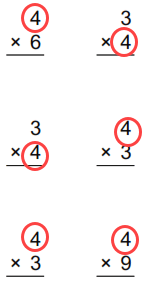Multiplying by 3
Multiplying by 4
Multiplying by 5
Multiplying by 6
Multiplying by 7
Multiplying by 8
Multiplying by 9
Multiplying by 10
Multiplying by 11
Multiplying by 12

## Focus numbers (pairs of numbers)

Multiply by 0 and 1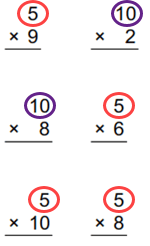Multiply by 2 and 3
Multiply by 4 and 6
Multiply by 5 and 10
Multiply by 7 and 8
Multiply by 3 and 9
Multiply by 11 and 12

## Focus numbers (groups of numbers)

Multiply by 2,3 or 4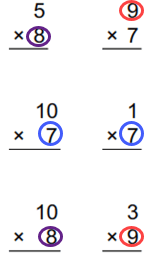Multiply by 2,5 or 10
Multiply by 3, 4 or 6
Multiply by 7,8 or 9
Multiply by 10,11 or 12

## Circle drills

Circle drills (multiplication) 1-10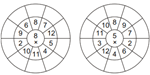Circle drills (multiplication) 2-12

## Missing factor questions

Missing factors up to 7 __ x 6 = 24
Missing factors 1-10 7 X __ = 63
Missing factors 1-12 11 X __ = 108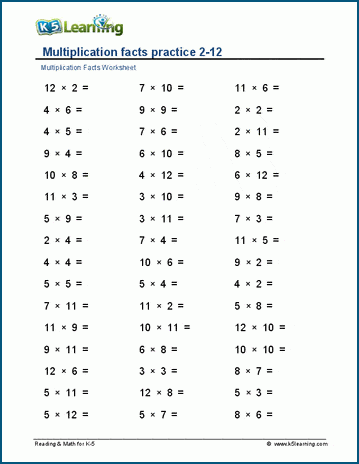Sample multiplication facts worksheet

What is K5?

K5 Learning offers free worksheets, flashcards and inexpensive workbooks for kids in kindergarten to grade 5. Become a member to access additional content and skip ads.